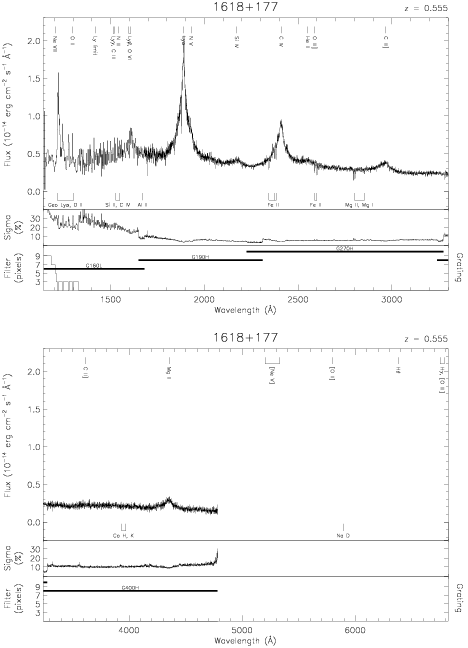Download dataset
TELESCOP= 'HST     '
INSTRUME= 'FOS     '
EQUINOX =               2000.0
DATE-OBS= '1991-09-07T01:10:12'
RA_PNT  =       245.0908333333
DEC_PNT =        17.6066628745
PA_PNT  =       309.5848074473
OBJECT  = '1618+177'
ROOTNM01= 'Y0PE0G02T'
GRNDMD01= 'SPECTROSCOPY'
DETECT01= 'AMBER   '
APERID01= 'A-1     '
FGWAID01= 'H19     '
ROOTNM02= 'Y0PE0G03T'
GRNDMD02= 'SPECTROSCOPY'
DETECT02= 'AMBER   '
APERID02= 'A-1     '
FGWAID02= 'H27     '
ROOTNM03= 'Y0PE0G04T'
GRNDMD03= 'SPECTROSCOPY'
DETECT03= 'AMBER   '
APERID03= 'A-1     '
FGWAID03= 'H40     '
ROOTNM04= 'Y0RV0E03T'
DETECT04= 'AMBER   '
APERID04= 'C-2     '
FGWAID04= 'H19     '
ROOTNM05= 'Y0RV0E04T'
DETECT05= 'AMBER   '
APERID05= 'C-2     '
FGWAID05= 'H19     '
ROOTNM06= 'Y0RV0E05T'